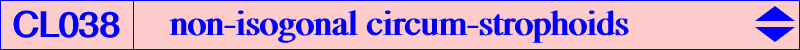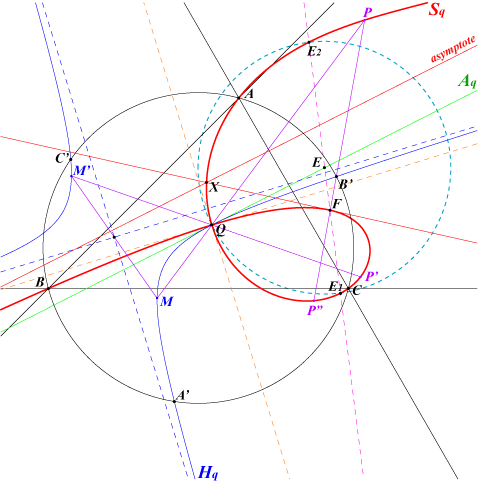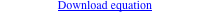CL003 is the class of isogonal circum-strophoids with node I, the incenter. Remember that these cubics form a pencil of focal nodal cubics. Similarly, it is possible to define three other pencils when I is replaced by an excenter. Given a point Q which is not an in/excenter, there is one and only one circum-strophoid Sq with node Q. Sq is the locus of point P such that the reflections of the cevian lines of P in the corresponding cevian lines of Q are concurrent at P', which is also a point on Sq, called the conjugate of P on Sq. All the known properties of strophoids are easily adapted in this configuration and are summarized below : Sq is the inversive image (in a circle centered at Q) of the rectangular hyperbola Hq passing through Q, the inverses A', B', C' of A, B, C and the orthocenter H' of A'B'C'. Notice that this does not depend of the radius of the circle, hence it is convenient to use the inversion with pole Q which swaps the vertices of ABC and those of the circumcevian triangle A'B'C' of Q. See figure below. Hq also contains the isogonal conjugate of the infinite point of a perpendicular to the line QQ* (Q* isogonal conjugate of Q) which is its fourth intersection with the circumcircle. The inverse of this point is the "last" point of Sq on the circumcircle. The inverses of the two points P and P' are two antipodes M and M' on Hq. In particular, the inverse of the antipode Q' of Q is the singular focus F of Sq. The circle QB'C' meets Hq in a fourth point whose inverse is the third point of Sq on BC. The intersections with CA and AB are defined similarly. The real asymptote is parallel to the tangent at Q to Hq and passes through the reflection of F in Q. Obviously, the nodal tangents at Q to Sq are parallel to the asymptotes of Hq. Sq meets its asymptote at X lying on the perpendicular at Q to FQ and FX is the focal tangent. FX is also the reflection of Aq in the perpendicular bisector of FQ. The perpendicular at F to FQ meets the axis Aq of the strophoid (i.e. the tangent at Q to Hq) at E and the circle with center E passing through Q meets Sq at E1, E2 centers of anallagmaty of Sq. These points E1, E2 also lie on the bisectors of the nodal tangents and on the perpendicular at F to FQ and, naturally, the tangents at E1, E2 to Sq are parallel to the real asymptote. The polar conic of F in Sq is the circle Cq through F and Q which is centered on the normal at F to Sq. Cq also contains the intersections of the perpendicular bisector of FQ with the nodal tangents. The line FP meets Cq again at P1 and the harmonic conjugate of P with respect to F and P1 is a point P" on Sq. More precisely, since P' is the conjugate of P on Sq, the line P'P" is parallel to the asymptote and the nodal tangents of the strophoid bisect the angle (QP,QP'). The midpoint of P'P" lies on the polar conic of the real infinite point of Sq. This is the rectangular hyperbola passing through Q, E1, E2 having one asymptote that of Sq.The equations of Sq and Hq are rather complicated and you may want to download a text file.Additional remarks : Hq meets the circumcircle again at a point whose inverse is the "last" intersection of Sq with the circumcircle. Sq has only one real inflexion point J lying on the homothetic of E1E2 under h(Q,2). J is the inverse of the antipode on Hq of the foot T of the normal dropped from Q to Hq. T lies on the Apollonian hyperbola of Q with respect to Hq. This is the rectangular hyperbola passing through Q, the center W of Hq, the projections of Q on the asymptotes of Hq. Its asymptotes are parallel to the bisectors of the nodal tangents at the midpoint of WQ. It is not possible to construct T with ruler and compasses only.Now, if Q and R are two (distinct) isogonal conjugates, the corresponding strophoids Sq and Sr are themselves isogonal conjugates and their foci Fq, Fr are inverse in the circumcircle. Examples • with Q = X(4) and R = X(3), we obtain the Ehrmann strophoid K025 and the Jerabek strophoid K039 respectively with foci X(265) and its inverse X(5961). • with X(36) and X(80), we find the two strophoids K274 and K275. • with X(2) and X(6), we find the two strophoids K1298 and K1299. See also the Fermat strophoids K061-a and K061-b. Remarks — Sq is a K0 if and only if Q lies on Q039. — Sq contains O if and only if Q lies on Q023. — Sq contains H if and only if Q lies on Q038. — Sq contains I if and only if Q lies on Q181.